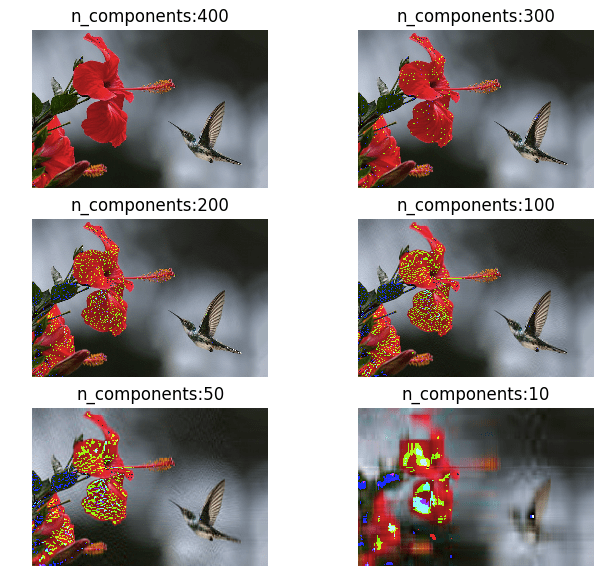# Statistics## Otsu’s method for image thresholding explained and implemented

The process of separating the foreground pixels from the background is called thresholding. There are many ways of achieving optimal thresholding and one of the ways is called the Otsu’s method, proposed by Nobuyuki Otsu. Otsu’s method is a variance-based technique to find the threshold value where the weighted variance between the foreground and background …

## Linear Regression using Gradient Descent Algorithm

Gradient descent is an optimization method used to find the minimum value of a function by iteratively updating the parameters of the function. Parameters refer to coefficients in Linear Regression and weights in Neural Networks. In a linear regression problem, we find a modal that gives an approximate representation of our dataset. In the below …## Mathematics of Principal component analysis

Principal component analysis is a method used to reduce the number of dimensions in a dataset without losing much information. It’s used in many fields such as face recognition and image compression, and is a common technique for finding patterns in data and also in the visualization of higher dimensional data. PCA is all about …

## Evaluating the fitness of a modal with a cost function

Previously we derived a simple linear regression modal for our Pizza price dataset. We built a modal that predicted a price of $13.68 for a 12 inch pizza. When the same modal is used to predict the price of an 8 inch pizza, we get$9.78 which is around $0.78 more than the known price of$9. …

## Math behind Linear Regression with Python code

Simple linear regression is a statistical method you can use to study relationships between two continuous (quantitative) variables: independent variable (x) – also referred to as predictor or explanatory variable dependant variable (y) – also referred to as response or outcome The goal of any regression model is to predict the value of y (dependant variable) based on the …

## Understanding Binomial Distribution using Python

Binomial distribution is used to understand the probability of a particular outcome in repeated independent trials. The probability of a trial is either success or failure. The trials are independent as the outcome or the previous trial had no effect on the next trial, as happens in tossing of coins. If we flip a coin, it would either …

## Poisson distribution with Python

A Poisson distribution is the probability distribution of independent occurrences in an interval. Poisson distribution is used for count-based distributions where these events happen with a known average rate and independently of the time since the last event. For example, If the average number of cars that cross a particular street in a day is 25, …

## Markow chain explained in simple words

Markow chain is a probabilistic process used to predict the next step based on the probabilities of the existing related states. Its called a chain because the probability of the next step is dependant on the other steps in the group. For example, if the weather is cloudy then its highly likely that it might rain (The next …